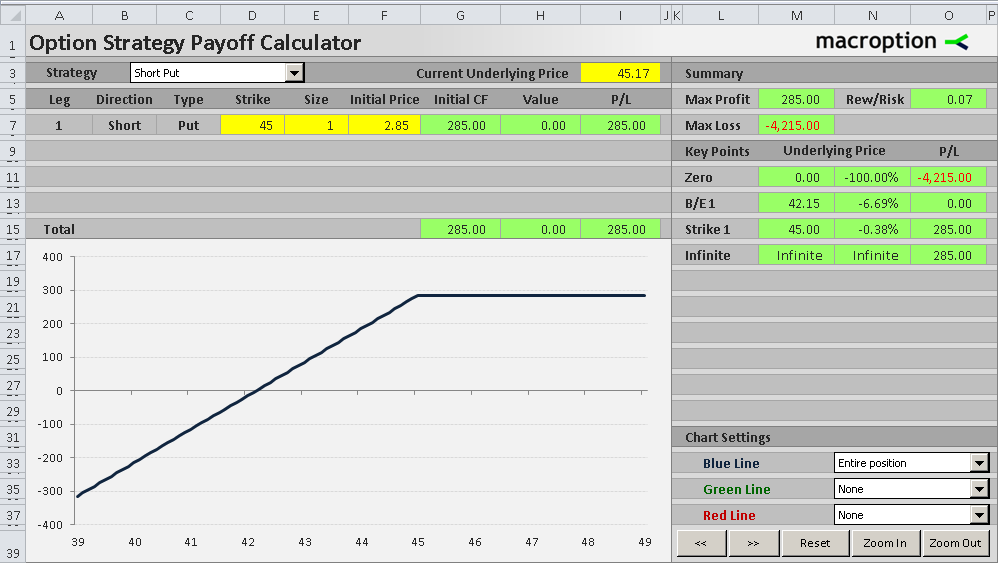# Short Put Payoff Diagram and Formula

This page explains short put option payoff. You can find similar pages for the other basic option positions here: long call payoff, short call payoff, long put payoff.

## Short Put Payoff Diagram

A short put option position is a bullish strategy with limited upside and limited (but usually very high) risk. The position is initiated by selling a put option with the intention to buy it back later at a lower price or waiting until expiration and hoping it will expire out of the money. See the payoff chart below:The payoff is inverse of long put position, which is the other side of your trade. Maximum profit is reached when the underlying security ends up at or above the put option's strike price and the option expires worthless. Below the strike price your profit declines in proportion with the underlying price.

## Best and Worst Case Scenarios

Let's use the example from the screenshot above to explain the best and worst case scenario for a short put strategy. In this example we have sold one contract of a 45 strike put option for the price of 2.85 per share, which is \$285 for one option contract representing 100 shares.

### Maximum Profit

The maximum you can gain from a short put trade is the amount you receive at the beginning when selling the put. If the option expires worthless, there is no more cash flow from the trade and you keep all the initial cash, which is also your total profit. For this to happen, the underlying security must end up at or above the put option's strike price at expiration.

In our example, the maximum possible gain is \$2.85 per share, or \$285 for one option contract.

### Maximum Loss

The worst case scenario is when the underlying price drops to zero. The put option is exercised, which makes you as the option seller buy the underlying at the option's strike price. Because the underlying is now worthless, you lose the amount equal to the strike price (per share). Total loss from the trade is therefore equal to the strike price less the initial amount you have received when selling the put.

In our example the maximum possible loss is equal to the strike price (\$45) less the initial option price (\$2.85), which is \$42.15 per share, or \$4,215 for one option contract representing 100 shares.

## Risk-Reward Ratio

The risk-reward ratio is usually quite unfavorable with a short put position, as the maximum possible loss is usually much higher than potential profit of the trade.

In our example the risk (\$4,215) is almost 15x greater than the potential gain (\$285).

## Short Put Payoff Formulas

If you have seen the explanation of long put option payoff formulas, you will find the short put payoff formulas are exactly the same, only with opposite signs, as you are now taking the other side of the trade.

There are again two components of the total profit or loss:

• The initial option price
• The value of the option at expiration

Short put payoff per share = initial option price – option value at expiration

Put option value at expiration = MAX(0, strike price – underlying price)

Short put payoff per share = initial option price – MAX(0, strike price – underlying price)

Short put payoff = (initial option price – MAX(0, strike price – underlying price)) x number of contracts x contract multiplier

## Break-Even Point

The break-even point of a short put position is exactly the same as long put break-even.

Short put B/E = strike price – initial option price

Using the same example as above, strike price is \$45 and initial option price is \$2.85, which makes the break-even equal to

45 – 2.85 = \$42.15

This particular short put trade is profitable if the underlying ends up above 42.15; if ends up below this price, the trade will be a loss. You can also see it in the chart, where the P/L line crosses the zero line at 42.15.## Summary

• Short put strategy is directional and bullish. It is generally profitable when the underlying price goes up (or doesn't go down at least).
• It is also a short volatility strategy, as the value of a put option declines when volatility decreases, which means your short put position becomes more profitable.
• In other words, when selling a put option, you want the underlying security to not go down, or not move at all (assuming you've sold an out of the money put). You want the underlying price to end up above the strike price, so the put option expires worthless and you keep the entire premium.
• Short put strategy has limited upside, equal to the cash you get when selling the put option in the beginning. This is the maximum you can gain from the trade.
• It has limited risk (unlike a short call trade whose risk is unlimited), equal to the strike price less the initial option price. However, in most cases the option price is much lower than the strike price, which means the maximum possible loss is typically much higher than the potential profit.

By remaining on this website or using its content, you confirm that you have read and agree with the Terms of Use Agreement.

We are not liable for any damages resulting from using this website. Any information may be inaccurate or incomplete. See full Limitation of Liability.

Content may include affiliate links, which means we may earn commission if you buy on the linked website. See full Affiliate and Referral Disclosure.

We use cookies and similar technology to improve user experience and analyze traffic. See full Cookie Policy.